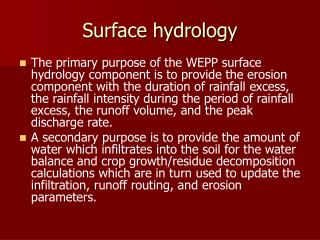DownloadDownload PresentationSurface hydrology

# Surface hydrology

Télécharger la présentation## Surface hydrology

- - - - - - - - - - - - - - - - - - - - - - - - - - - E N D - - - - - - - - - - - - - - - - - - - - - - - - - - -
##### Presentation Transcript

1. Surface hydrology • The primary purpose of the WEPP surface hydrology component is to provide the erosion component with the duration of rainfall excess, the rainfall intensity during the period of rainfall excess, the runoff volume, and the peak discharge rate. • A secondary purpose is to provide the amount of water which infiltrates into the soil for the water balance and crop growth/residue decomposition calculations which are in turn used to update the infiltration, runoff routing, and erosion parameters.

2. Spatially, the program predicts detachment and deposition at each of a minimum of 100 points on a hillslope, and the sum totals of these values are divided by the number of years of simulation in order to have average data at each point.

3. Processes • The sequence of calculations relevant to surface hydrology are infiltration, rainfall excess, depression storage, and peak discharge • Infiltration is computed using an implementation of the Green- Ampt Mein-Larson model for unsteady intermittent rainfall.

4. Green and ampt infiltration • The Green-Ampt model is the first physically-based equation describing the infiltration of water into a soil. • This model yields cumulative infiltration and infiltration rates as implicit functions of time • The model is a lot more complex than the simple infiltration function but is more of a water balance infiltration function instead of a simple time power function.

5. Green and Ampt model • f= f*Kn where f is infiltration rate cm/hr • Kn=hydraulic conductivity • f*=(F+1)/(F*+z*) dimensionless infiltration • F*- ½{t*-2z*+ ((t*-2z*)^2+8t*)^0.5} • Where F* is the dimensionless accumulated infiltration in layer n where the wetting front is located. • z* is the dimensionless depth accounting for thickness and conductivity of layers behind the wetting front. Layers i to n-1

6. t*= Kn t/ ( delt O ( Hn+ ∑zi) • Where delt O is the change in volumetric water content as the wetting front passes layer n. • Hn is the potential head while the wetting front passes through layer n • Zi is the thickness and should be summed from i-1 to n-1 • Zi= the thickness of the layer .

7. Z*= Kn/ (Hn+ ∑zi) * ∑zi/Ki • Where ki is the hydraulic conductivity of layer i. • Kn= hydraulic conductivity of layer n containing the wetting front. • Hn is the potential head • http://weather.nmsu.edu/teaching_Material/soil470/green-ampp-inf.pdf

8. Surface hydrology • Overland flow processes are conceptualized as a mixture of broad sheet flow occurring in interrill areas and concentrated flow in rill areas. Broad sheet flow on an idealized surface is assumed for overland flow routing and hydrograph development. • Overland flow routing procedures include both an • analytical solution to the kinematic wave equations and regression equations derived from the kinematicapproximation for a range of slope steepness and lengths, friction factors (surface roughness coefficients),soil textural classes, and rainfall distributions.

9. Mannings equation is combined with the kinematic wave equations to compute velocity • v = 1/n x R^2/3 x S^1/2 • Where : • n = coefficient of roughness (typically 0.3) • v = Water velocity down the channel (m / sec) • R = Hydraulic radius (m) = cross sectional area (m2) / wetted perimeter (m) • S = Gradient of channel (m / 100m)

10. kinematic wave equations is the continuity equation • http://doctorflood.rice.edu/ceve101/Handouts/Ch04a.ppt • Simple continuity equation is I-0=del S/t • Where i=inflow • O= outflow • Del S is the change in storage and t is time. • Simple solutions to the deferential equation involve the muskingum method that makes simplifying assumptions

11. Differential equation for kinematic-flood-routing • http://www.alanasmith.com/theory-Kinematic-Flood-Routing.htm • http://www.webs1.uidaho.edu/ch/presentations/kw_applications.ppt#259,4,Kinematic Waves • http://www.webs1.uidaho.edu/ch/presentations/kw_applications.ppt#262,7,Kinematic Waves

12. Curve Number runoff • http://www.ecn.purdue.edu/runoff/documentation/scs.htm